Two rooms

Woman cleans the room in 30 minutes a man in 90 minutes. How long will take they clean two rooms?

Result

t =  45 min

Solution:

t/30+t/90 = 2

4t = 180

t = 45

Calculated by our simple equation calculator.

Leave us a comment of this math problem and its solution (i.e. if it is still somewhat unclear...):Be the first to comment!To solve this verbal math problem are needed these knowledge from mathematics:

Looking for help with calculating harmonic mean? Looking for a statistical calculator? Need help calculate sum, simplify or multiply fractions? Try our fraction calculator. Do you have a linear equation or system of equations and looking for its solution? Or do you have quadratic equation?

Next similar math problems:

1. PlayingHow long have we trained on the pitch when we know that the warm-up took 10 minutes, we trained passes for one-third of the time and we played football half the time?
2. Workers 2Worker dug the trench for 7 hours, another worker for 10 hours. They recruited to work even one worker more. Digging should be done for 2 hours. For how long would dig third worker alone?
3. NovakNovak needed to dig up three of the same pit in the garden. The first pit dug father alone for 15 hours. His second dig son helped him and it did that in six hours. The third pit dug son himself. How long it took him?
4. Painters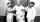One painter could paint a school for 7 days. The second one for 6 days. It was necessary to paint the school in two days. Next painter was invited and all worked together. How long would this painter painted the school itself?
5. PumpingThe tank is filled with a larger pump in 12 hours and with scond pump in 15 hours. For how long does the tank fill when both pumps are switched on at the same time?
6. Two tributaries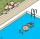Two tributaries of the pool fill it in 10 hours. One of the tributaries would fill 15 hours. How long would fill the first tributary?
7. Apprentice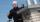Worker and apprentice execute the work for 6 hours. Worker performs himself in 10 hours. How long do the work of an apprentice?
8. Working together on projectTwo employees working on the project. First make it alone for 20 days the second for 24 days. How many days will take to complete project if 5 days they work together then first will take two days holiday and after returning they completes a project toget
9. Six yearsIn six years Jan will be twice as old as he was six years ago. How old is he?
10. Tanker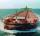An oil tanker can be emptied by the first pump in 4.9 hours. Second pump can empty an tanker in 8 hours. If the first pump started at 4:00 and second 1.4 hour later, at what time will the tanker be empty?
11. Three peopleThree people start doing a work at a same time. The first worked only 2 hours. The second ended 3 hours before the end. On an individual basis, it would take the first time to do the work 10 hours, second 12 hours and 15 hours third. How many hours did it.
12. Tonic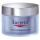One package tonic consume Mom 12 weeks and daughter for 14 weeks. 4 weeks they use the same cream together and then only daughter. How many weeks endured tonic to daughter then?
13. CandyPeter had a sachet of candy. He wanted to share with his friends. If he gave them 30 candies, he would have 62 candies. If he gave them 40 candies, he would miss 8 candies. How many friends did Peter have?
14. Bag of peanuts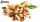Joe eat 1/3 of a bag of peanuts, mark eat 1/4 of the remaining in the bag of peanuts, Alvin eat 1/2 of the remaining bag of peanuts, peter eat 10 peanuts, there are 71 peanuts left. Hon many peanuts were in the bags?
15. Equation with xSolve the following equation: 2x- (8x + 1) - (x + 2) / 5 = 9
16. Fraction + eqSolve following simple equation with fractions: -5/6(8+5b) = 75 + 5/3b
17. Roses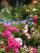On the large rosary was a third white, half red, yellow quarter and six pink. How many roses was in the rosary?